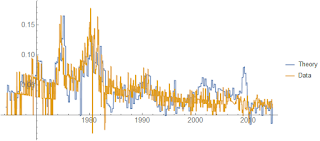## Wednesday, March 29, 2017

### "Improved" quantity theory of labor and capital

I added the modification from this post to the "quantity theory of labor and capital" (QTLK, aka the "nominal Solow model" without TFP dynamics) to the information equilibrium GitHub repository. The Mathematica notebook starts from the basic labor model of the first link, shows Okun's law, adds capital to obtain the QTLK of the second link, and then finally shows the "improved" QTLK described below.

In the information equilibrium notation the modified model is:

P : NGDP ⇄ L
NGDP ⇄ K

CPI ⇄ P

The modification is essentially changing the abstract price/detector in the first relationship to an unknown quantity P and adding the relationship CPI ⇄ P (that is to say we don't measure the abstract price P directly, but rather just something ‒ e.g. core CPI ‒ that is in information equilibrium with the abstract price).

The result is primarily a much improved description of inflation:Nominal growthcore CPI inflationcore CPI-deflated real growth

PS Why do I call this a "quantity theory of labor and capital"? Because it started out as a simple model of labor where (solving the differential equation described by the relationship P : NGDP ⇄ L)

log NGDP ~ α log L
log P ~ (α − 1) log L

Therefore if α = 2, P ~ exp π t, and L ~ exp λ t, we can say π = λ. That is to say inflation is equal to the growth rate of the (employed) labor supply. This is directly analogous to the quantity theory of money where inflation is equal to the growth rate of the money supply.

However, in practice α < 2. What we end up with is something more like the Solow model where:

log NGDP α log L + β log K
log ~ (α − 1) log L

And in the modified version we substitute the latter equation with

log ~ γ (α − 1) log L